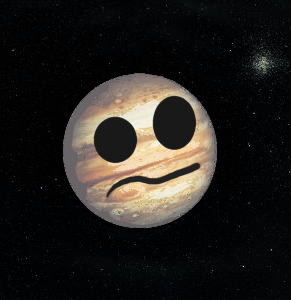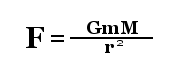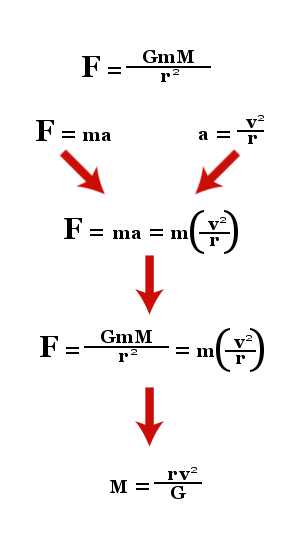## Sunday, March 27, 2011

### Spaced Out

I'm afraid the skunk cabbage seminar I promised last week has been postponed. Not only did a member of the peanut gallery neglect to use spoiler tags and COMPLETELY RUINED IT FOR EVERYBODY, but the skunk cabbage blog post is traditionally a celebration of the changing seasons, and after this last sucker punch from Old Man Winter I see precious little worth celebrating. Guess this means we'll have to think of another topic for our weekly heart to heart.

Sometimes when I chat with my father about a new astronomical discovery related in some popular science periodical or other, the old man will remark "seriously, how do they know any of this?" It's a sentiment I can appreciate, especially where science news for the layman is concerned. (This is certainly not to suggest that I am much more than a layman.) We'll read an article on livescience.com about the latest pulsar observations or speculations about dark energy, and the journalist, writing for a general audience, will skip right to the researchers' announcements without going into much detail about the recondite theories, postulates, observations, graphs, stacks of photographs, and walls of calculus from which the scientists produced their new discovery or hypothesis. Incredulous minds like my old man are reluctant to accept the validity of a statement when they can't see the reasoning on which it is based. After all, "because scientists say so" is just as vacuous and dangerous a position to take as "because the Church says so."

Today, in part fifty-seven-point-three-three-three of our ongoing Amateur Astronomy Series (tremendous emphasis on the "amateur " part), we will learn what scientists have deduced about the physical characteristics of a distant object, and more importantly, how they went about doing it. I would like to avoid turning this into another "LOOK AT SPACE AND HOW BIG AND CRAZY AND MINDBLOWING IT IS" piece: I would rather draw attention to the ingenuity the human mind has employed in coming to a more thorough understanding of the universe beyond our little blue pebblet, and in decrypting the code of the cosmic program.

What we're gonna do today is calculate the average density of the planet Jupiter.Jupiter

Density, as you know, is a measure of how much matter is pressed into a given space. Or to put it more simply:

mass (kg) / volume (m^3) = density (kg/m^3)

So in order to even begin doing this, we need to figure out Jupiter's volume and mass first. Both can be done without too much difficulty, especially since the real scientists have already done all the painstaking measurements and guesswork for us.

Let's begin with the planet's volume. Jupiter isn't quite spherical, but we're going to assume that it is, since I sure as hell don't know how to correct an equation to account for rotational flattening. Anyway, as you surely remember from sophomore geometry class (and as I just looked up on Wikipedia), the volume of a sphere can be calculated like so:

(4/3)πr^3

Great! But what the hell is Jupiter's radius?

If you recall from our last scintillating episode, astronomers have long known how to get a very close approximation of a distant object's size using basic geometry. For this, we're going to assume that the Earth and Jupiter are positioned roughly at their closest point of approach (which we'll talk more about in a minute). At this distance, Jupiter's angular diameter in the night sky is 46.8 arc seconds, which comes to about 0.013°. Now we plug it into this familiar equation...

(angular size)° / 360° = (Jupiter's diameter) km / 2π(Jupiter's distance from Earth) km

And we get...

0.013° / 360° = (Jupiter's diameter) km / 2π(Jupiter's distance from Earth) km

Wait. That's no good. We still need to figure out Jupiter's distance from Earth. To do this, we turn to Mr. Johannes Kepler.Johannes Kepler

Thanks to the trove of meticulously-compiled stellar records left to him by Tycho Brahe and the eighteen years he spent poring over them, crunching numbers, and making observations of his own, Kepler discovered that the planets' orbits obey three basic rules.

Kepler's first law states that planetary orbits are elliptical. Think of an ellipse as a circle with two centers (called foci). In a circle, every point on the circumference is equidistant from the center. With an ellipse, you can pick any point on the curve, measure the distances between it and both foci, add them together, and come to the same sum you would with any other point on the curve.

Anyhow. When considering planetary distances from the sun, standard procedure is to use the semimajor axis, which really just means "one half of the ellipse's width." Kepler assigns Earth's semimajor axis the value of 1 astronomical unit (A.U.).

Kepler's third law establishes the relationship between a planet's distance from the sun and the times it takes to complete one orbital revolution. Put simply:

(orbital period in Earth years)^2 = (semimajor axis in A.U.)^3

Kepler then calculated and published the orbital periods and semimajor axes of the first six planets. Thanks to him, we know that Jupiter has a semimajor axis of 5.2 A.U. Since Earth's semimajor axis is 1 A.U., we're going to assume that Earth and Jupiter are positioned so that the distance between them is roughly 4.2 A.U. (which, again, is about where they are during their closest approach).

But wait: this doesn't do us much good either. We're not looking for a measurement in astronomical units. We need kilometers.

Before the 20th Century, astronomers used triangulation to arrive at an approximation of the distance between Earth and Venus. Since Earth's semimajor axis is 1 A.U., and Venus's semimajor axis is about 0.7 A.U., they reckoned that the difference between Earth and Venus (in kilometers) would correspond to roughly 0.3 A.U. From there, they could use that value to determine how many kilometers are in one astronomical unit. Since Venus is about 45,000,000 km away from the Earth, 0.3 A.U. equates to 45,000,000 km. Dividing the latter figure by the former gives us 150,000,000 km for 1 A.U. (Scientists experimenting with radar in the 20th Century pegged the value at a more precise 149,597,870 km, but 150,000,000 km is still used for simplicity's sake.)

So! If Jupiter's distance from Earth is 4.2 A.U., and an A.U. is equal to 1.5 x 10^8 km, then Jupiter's distance from the Earth in kilometers is 6.3 x 10^8 (or 630,000, 000).

Back to the equation!

0.013° / 360° = (Jupiter's diameter) km / 2π(630,000,000) km

What we end up with is some huge number with a lot of decimal places that we're just gonna call 143,000 km. So if that's Jupiter's diameter, that means its radius is about 71,500 km. Now, at last, we can figure out its volume:

(4/3)π(71,500)^3 = 1,531,111,204,795,974.7523

Whoa! Let's just round that down to a much more manageable 1,530,000,000,000,000 km^3. And since density (which is what we're ultimately trying to determine, remember?) more often uses meters than kilometers, let's convert it into meters for a final value of:

1,530,000,000,000,000,000,000,000 m^3 = Jupiter's volume

Still with me? I will be inconsolably heartbroken if you are not.

Now that we know how much space Jupiter occupies, we still have to figure out how massive it actually is. I saved this part for last because I think it is very cool. First, let's look at Jupiter's moon Europa.Europa

This icy little fella revolves around Jupiter in a circular orbit that takes 3.55 days to complete and whose angular radius appears as 3.66 arc minutes (0.061° ).

First, we'll calculate the circumference of Europa's orbit. We get its radius through the same trick we used to find Jupiter's diameter, arriving at about 671,000 km. From here, finding the circumference is as easy as:

2π(671,000) km = 4,216,017.341 km

We'll round that up to 4,220,000 km since we're too cool to give a damn. But you wonder: where are we going with this?

So we know Europa travels a distance of 4,200,000 km over a period of about 3.55 days. Now we're going to smash these values together to determine its speed. Since speed is usually more concerned with "per second" than "per day," we convert 3.55 days into about 307,000 seconds. So if Europa moves 4,200,000 kilometers every 307,000 seconds, then its speed must be somewhere in the neighborhood of 13.7 km/s.

Now the ball enters the court of Sir Issac Newton.Sir Issac Noo-Tun

Europa maintains a speed of 13.7 km/s as it orbits Jupiter. Its velocity, however, constantly changes. Speed only concerns itself with distance and time; velocity also considers direction. By Newton's definitions, since Europa moves in a circle instead of a straight line, the moon is accelerating.
Newton determined that the acceleration of any body moving at speed v in a circular orbit of radius r may be shown to be:

a = v^2 / r

Using this equation (and remembering that Europa's orbital radius is half of its orbital diameter), we find that Europa accelerates at a rate of about 0.00056 km per second per second.

According to Newton's laws of motion, an object accelerates when a force is applied to it. In Europa's case, that force is the gravitational interaction between it and Jupiter. Here is how we calculate gravitational force:Let's take a moment to define these terms. F represents force, measured in newtons (N). M and m represent the respective masses of the larger and smaller bodies (in this case, Jupiter and Europa) in kilograms (kg). r represents the distance between bodies M and m (in other words, the orbital radius) in meters (m). And G stands for the gravitational constant of proportionality and has a set value of 6.67 x 10^-11 n m^2/kg^2.

This is where it gets wacky and brilliant. We can determine how much gravitational force Jupiter exerts on Europa based on the moon's acceleration. Then we can figure out Jupiter's mass from the amount of gravitational force it exerts on Europa. The key to doing this is lies in Netwon's second law of motion: force equals mass times acceleration, or...

F = ma

Let's go over this one more time. We've got force (F), measured in newtons (N). We've got Europa's mass (m) and Jupiter's mass (M), measured in kilograms (kg). We've got Europa's orbital radius (r) measured in meters (m). We've got Europa's orbital speed (v) and orbital acceleration (a), measured in meters per second (m/s) and meters per second per second (m/s^2), respectively. And finally, we have the gravitational constant (G), which is 6.67 x 10^-11 n m^2/kg^2.

What you are about to see is some serious algebraic ninjutsu, courtesy of Sir Issac Newton. Don't blink or you'll miss it:Swish!

All that's left is to plug in the values and solve. (Remember: even though we used kilometers for Europa's orbital radius and speed, we're converting them to meters now.)

M = (671,000,000 x 13,700^2) / 6.67 x 10^-11

And so, we get:

M = 1.9 x 10^27 kg

Or:

M = 1,900,000,000,000,000,000,000,000,000 kg

And so, having determined Jupiter's mass and volume, we can at last figure out its average density:

1.9 x 10^27 kg / 1.53 x 10^24 m^3 = 1.242 x 10^3 kg/m^3

Jupiter's average density would appear to be 1,242 kilograms per cubic meter by our reckoning. According to NASA, Jupiter's average density is actually more like 1,326 kilograms per cubic meter, but remember: they've corrected their equations to account for the planet's rotational flattening and use much more precise measurements than we did. But all in all, we came pretty close.

As far as the accuracy of these methods and figures are concerned, you need look no further than the unmanned probes that have visited Jupiter over the last thirty years for verification. It would be pretty hard getting a satellite in orbit around a planet 400,000,000 miles away without having a damned good idea of its mass and gravitational force in advance. And do note that these same probes were what ultimately confirmed the old "Jupiter is a gaseous planet" hypothesis, which was originally established on the grounds of the same average density calculations we just performed.

1.My first true "Ah ha!" moment in realizing just how big the universe is was when I discovered that all of planet Earth could fit into the "red spot" on Jupiter anywhere from one to three times.

Later on I saw that series of pictures on "Just how insignificant the Earth is in the universe" and then I went home and stared at the wall for an hour.

2.ಠ_ಠ WTF happened to my last comment? I hate blogspot.

I recommend everyone read this: http://scholarcitizen.williamcronon.net/

Also, I replied to you.

3.This seems like it would be relevant to you: http://www.techradar.com/news/internet/26-year-old-becomes-millionaire-self-publishing-on-kindle-store-931963

4.ADAM: From my astro-text: "Indeed, Jupiter has more than twice the mass of all the other planets combined, and it is such a large planet that many celestial mechanicians -- those researchers concerned with the motions of interacting cosmic objects -- regard our solar system as having only two important objects: the Sun and Jupiter....[I]n the broadest sense, our solar system is a two-object system with a lot of additional debris."

Little humility never hurt anyone, though.

5.